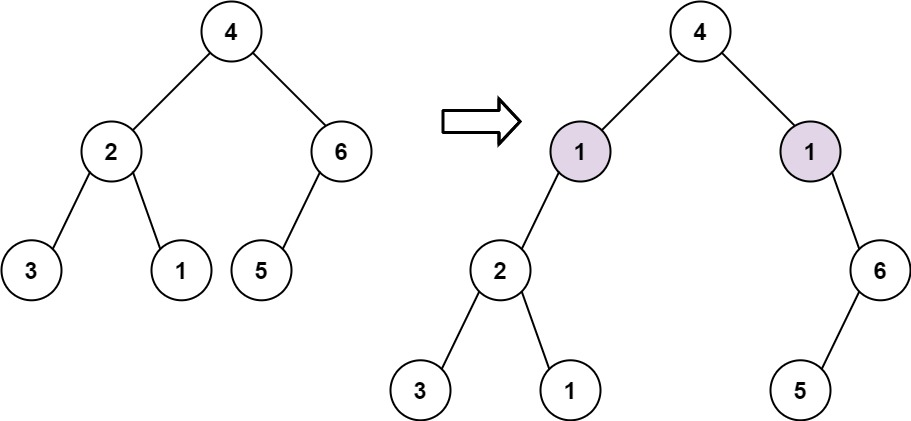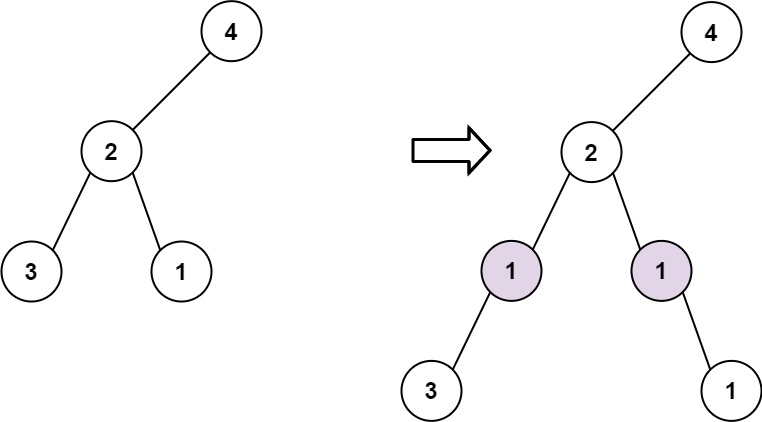GeetCode Hub

Given the root of a binary tree and two integers val and depth, add a row of nodes with value val at the given depth depth.

Note that the root node is at depth 1.

• Given the integer depth, for each not null tree node cur at the depth depth - 1, create two tree nodes with value val as cur's left subtree root and right subtree root.
• cur's original left subtree should be the left subtree of the new left subtree root.
• cur's original right subtree should be the right subtree of the new right subtree root.
• If depth == 1 that means there is no depth depth - 1 at all, then create a tree node with value val as the new root of the whole original tree, and the original tree is the new root's left subtree.

Example 1:Input: root = [4,2,6,3,1,5], val = 1, depth = 2
Output: [4,1,1,2,null,null,6,3,1,5]

Example 2:Input: root = [4,2,null,3,1], val = 1, depth = 3
Output: [4,2,null,1,1,3,null,null,1]

Constraints:

• The number of nodes in the tree is in the range [1, 104].
• The depth of the tree is in the range [1, 104].
• -100 <= Node.val <= 100
• -105 <= val <= 105
• 1 <= depth <= the depth of tree + 1

/** * Definition for a binary tree node. * public class TreeNode { * int val; * TreeNode left; * TreeNode right; * TreeNode() {} * TreeNode(int val) { this.val = val; } * TreeNode(int val, TreeNode left, TreeNode right) { * this.val = val; * this.left = left; * this.right = right; * } * } */ class Solution { public TreeNode addOneRow(TreeNode root, int val, int depth) { } }